"Cloudster" Construction Project Session #33

Speed 400  Cloudster Project

The validation for making a polyhedral wing instead of a dihedral wing on the Jim Adams Cloudster plans comes from the original Cleveland Cloudster plans shown below.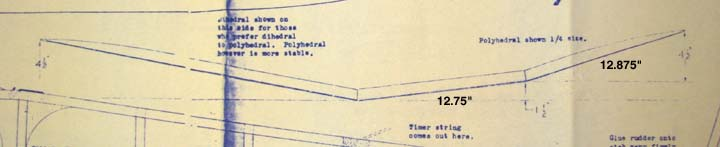This report deals with the issue of developing the polyhedral wing geometry for the Jim Adams Cloudster plans using the Cleveland Cloudster plans. To begin, the measurements and calculations for the polyhedral wing shown on the original Cleveland Cloudster plans are as follows:
Note:
Given:
Inner Panel Span (Li) = 12-3/4" (12.750")  measured
Tip Panel Span (Lt) = 12.-7/8" (12.875")    measured
Inner Panel Vertical rise = 1-1/2" (1.50")
Tip Panel Vertical rise = 4-1/2" (4.50")

1. Tip Span (Lt) to Semi-Span (b/2) Ratio = 0.5024 <<<<<<<

2. Inner Panel Dihedral Angle (d) = ArcSin (1.5/Li) = 6.756 Deg <<<<<<<

3. Angle (J) = ArcSin (4.5/Lt) = 20.458 Deg

4. Tip Panel Polyhedral Angle (c) = J-d = 13.701Deg <<<<<<<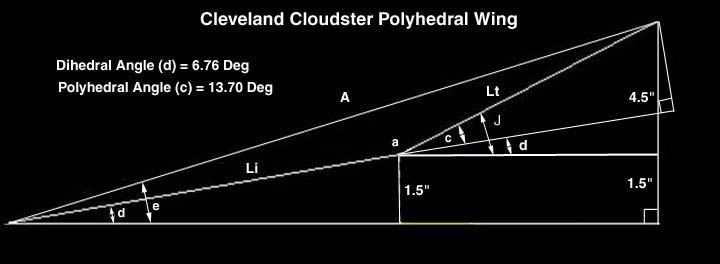Now, with the above geometry and wing data, the measurements and calculations used to development of the Jim Adams Cloudster polyhedral wing are as follows:
Notes:
1. The wing center section will remain flat over fuselage for ease of wing attachment.
2.The wing's inner and tip panel angles were made the same
on the Jim Adams plans as on the Cloudster plans.
Given:
Semi-span (b/2) = 23-15/16" (23.9375")  measured
1/2 Center section Span (1/2 Lc/s) = 1.223"  measured

Calculated Above:
Tip Span (Lt) to Semi-Span (b/2) Ratio = 0.5024
Inner Panel Dihedral Angle (d) = 6.756 Deg
Tip Panel Polyhedral Angle (c) = 13.701 Deg

1. Tip Span (Lt) = 0.5024 X 23.9375 = 12.027" <<<<<<<<

2. Inner Panel Span (Li') = 23.938-1.223-12.027 = 10.688" <<<<<<<< (measurement checks at 10-11/16")

Note:
This will require changing the wing's rib spacing to make
equal rib spacing with a rib falling at the polyhedral break.

3. New Rib Spacing = Li'/6 = 1.7813" <<<<<<<<

4. Inner Panel Vertical Rise (Hi') = Li' X Sin d = 1.257" <<<<<<<<

5. Tip Panel Vertical Rise (Ht) = Lt X Sin c = 2.849" <<<<<<<<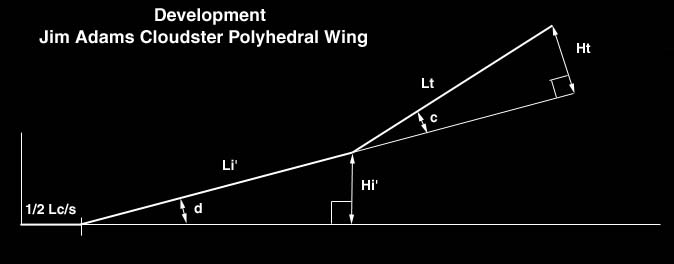Based on the 1.78" rib spacing of Item No. 3 above, The new rib locations were drawn in on the Jim Adams wing plan as shown below.
Inner Panel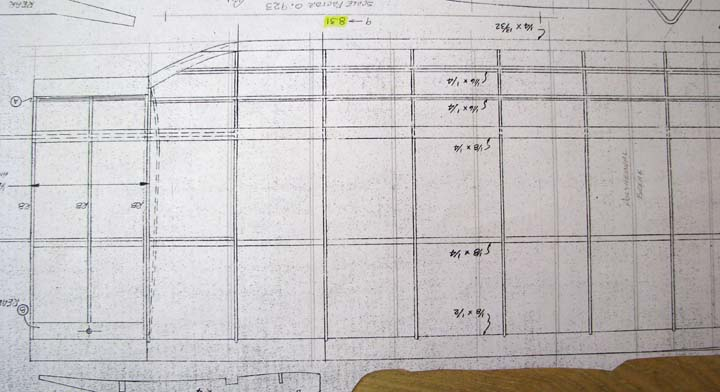Tip Panel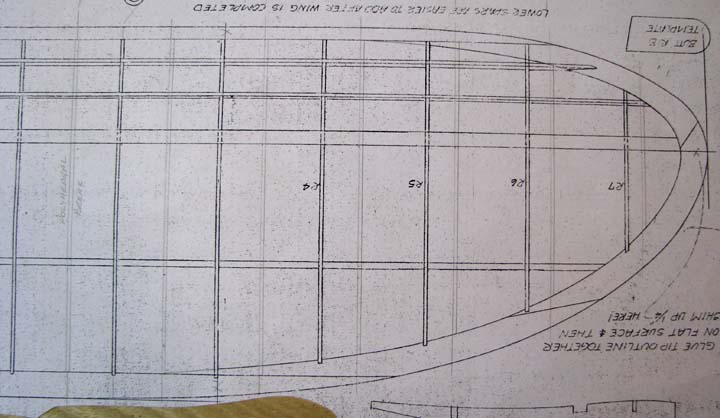The parts are available and the geometry has been calculated so that tomorrow the construction of the Jim Adams Cloudster polyhedral wing can begin..........................Tandy